# How to use the SUBTOTAL function in Excel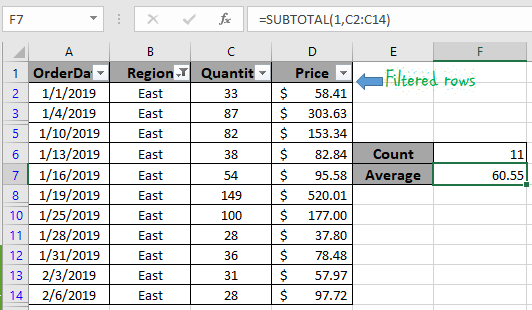In this article, we will learn How to use the SUBTOTAL function in Excel.

Obtain results on filtered rows

The biggest problem with SUM, AVERAGE, COUNT and other NUMBER functions is, that they include rows that are hidden by filter. They don’t work correctly when we want to sum, count, or do any other number task only on a visible cell. Here steps in the SUBTOTAL function of Excel.

SUBTOTAL Function Syntax :

 =SUBTOTAL(function_number, ref1, [ref2]...)

Function_number: Subtotal supports various number functions. To easily access them, it uses a function number. Below is the table of function number with corresponding functions.

 Function Number To Include Manually Hidden Rows Function Number To Ignore Manually Hidden Rows Function 1 101 AVERAGE 2 102 COUNT 3 103 COUNTA 4 104 MAX 5 105 MIN 6 106 PRODUCT 7 107 STDEV 8 108 STDEVP 9 109 SUM 10 110 VAR 11 111 VARP

Ref1 : The named range or reference of numbers.

[ref2] : optional named range or reference range to numbers.

Example :

All of these might be confusing to understand. Let's understand how to use the function using an example. Here we have this table of real estate. Now I want to add a total row but it should show me the sum of visible cells only when I apply a filter.

Write this formula in the end of table or the top. Your choice…

 =SUBTOTAL(9,C2:C16)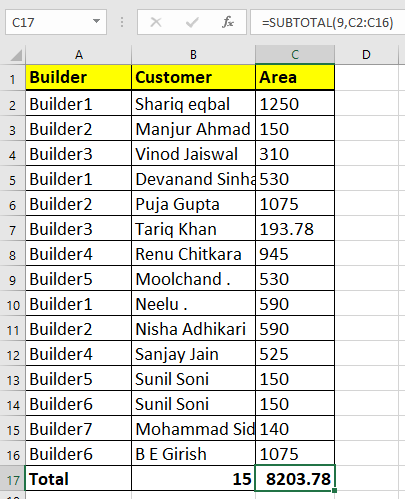Now whenever you will apply a filter, only visible cells total will be shown.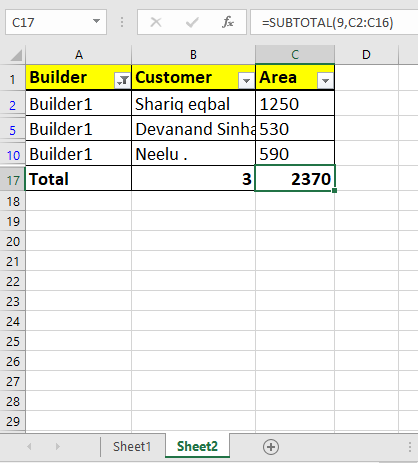As you can see from the above image the sum of total area of filtered rows (1250 + 530 + 590) comes out to be 2370. Isn't this impressive.

COUNT values on filtered rows

For this article we will be required to use the SUBTOTAL function. Now we will make a formula out of the function. Here we are given some values in a range and specific text value as criteria. We need to count the values where the formula includes all the values which ends with the given text or pattern

Generic formula:-

 = SUBTOTAL ( fun_num, range )

fun_num : number, num corresponding to the operation

range : range where operation is to apply.

Example:

All of these might be confusing to understand. So, let's test this formula via running it on the example shown below.

Here we have the order import data. And we have applied criteria to Region, only the East region is filtered.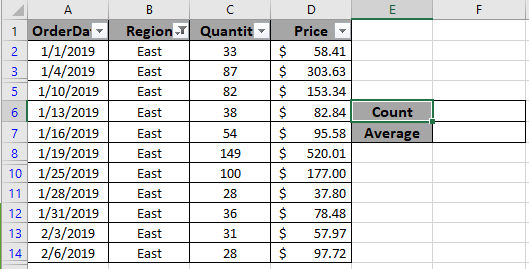Here to find the count of filtered values. Choose the right argument as fun_num. Fun_num is the operation you want to apply. Here to count the cells we use COUNTA operation, num as 3.

Use the formula:

 = SUBTOTAL ( 3, B2:B14)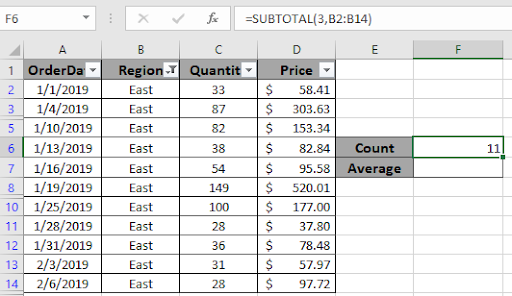As you can see the total rows which are visible comes out to be 11. Now we will use one more example to extract the Average of the quantity which are visible or filtered.

Use the formula:

 = SUBTOTAL ( 1 , C2:C14 )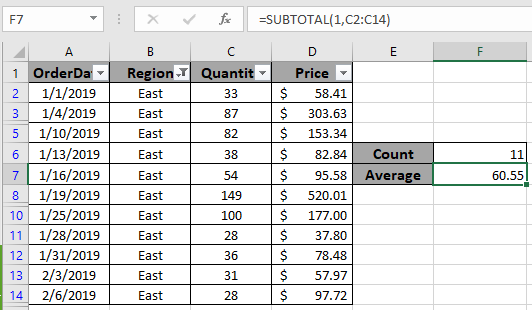As you can see the average order quantity received from the East region comes out to be approx 60. You can use different operations like average, count, max, min, product, standard deviation, sum or variation as per the required results on filtered data.

Here are some observational notes shown below.

Notes:

1. Choose the right fun_num to get the desired results.
2. The formula only works with numbers and text both
3. Operators like equals to ( = ), less than equal to ( <= ), greater than ( > ) or not equals to ( <> ) can be performed within functions applied on numbers only.

Hope this article about How to use the SUBTOTAL function in Excel is explanatory. Find more articles on calculating values and related Excel formulas here. If you liked our blogs, share it with your friends on Facebook. And also you can follow us on Twitter and Facebook. We would love to hear from you, do let us know how we can improve, complement or innovate our work and make it better for you. Write to us at info@exceltip.com.

Related Summing Articles :

How to use the SUMPRODUCT function in Excel: Returns the SUM after multiplication of values in multiple arrays in excel.

SUM if date is between : Returns the SUM of values between given dates or period in excel.

Sum if date is greater than given date: Returns the SUM of values after the given date or period in excel.

2 Ways to Sum by Month in Excel: Returns the SUM of values within a given specific month in excel.

How to Sum Multiple Columns with Condition: Returns the SUM of values across multiple columns having condition in excel

How to use wildcards in excel : Count cells matching phrases using the wildcards in excel.

Related Count Articles :

COUNTIFS with Dynamic Criteria Range : Count cells dependent on other cell values in Excel.

COUNTIFS Two Criteria Match : Count cells matching two different criteria on list in excel.

COUNTIFS With OR For Multiple Criteria : Count cells having multiple criteria match using the OR function.

The COUNTIFS Function in Excel : Count cells dependent on other cell values.

How to Use Countif in VBA in Microsoft Excel : Count cells using Visual Basic for Applications code.

How to use wildcards in excel : Count cells matching phrases using the wildcards in excel

Popular Articles :

How to use the IF Function in Excel : The IF statement in Excel checks the condition and returns a specific value if the condition is TRUE or returns another specific value if FALSE.

How to use the VLOOKUP Function in Excel : This is one of the most used and popular functions of excel that is used to lookup value from different ranges and sheets.

How to use the SUMIF Function in Excel : This is another dashboard essential function. This helps you sum up values on specific conditions.

How to use the COUNTIF Function in Excel : Count values with conditions using this amazing function. You don't need to filter your data to count specific values. Countif function is essential to prepare your dashboard.

Terms and Conditions of use

The applications/code on this site are distributed as is and without warranties or liability. In no event shall the owner of the copyrights, or the authors of the applications/code be liable for any loss of profit, any problems or any damage resulting from the use or evaluation of the applications/code.